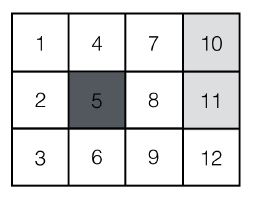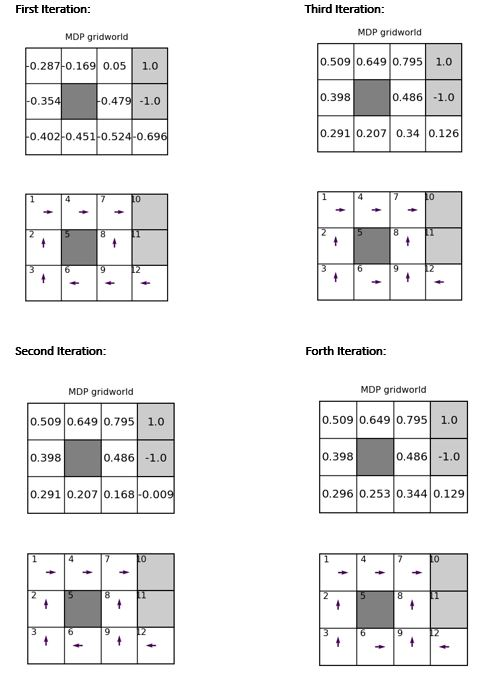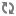## using markov decision process (MDP) to create a policy – hands on – python example

Hello there, i hope you got to read our reinforcement learning (RL) series, some of you have approached us and asked for an example of how you could use the power of RL to real life. for that reason we decided to create a small example using python which you could copy-paste and implement to your business cases. for us to move forward you have to make sure you know all the prerequisite needed to start using RL methodology, so for a quick recap go through this blog post we wrote a couple of months ago: https://g-stat.com/reinforcement-learning-getting-the-basics-series/.

Just a quick reminder, MDP, which we will implement, is a discrete time stochastic control process. It provides a mathematical framework for modeling decision making in situations where outcomes are partly random and partly under the control of a decision maker. Markov Decision Processes are a tool for modeling sequential decision-making problems where a decision maker interacts with the environment in a sequential fashion.

So, the problem we have in front of us goes like this, we have a world of 12 states, 1 obstacle initial state (state 5) and an 2 end states (states 10, 11). for each state we have a reward, we want to find the policy to implement for best reward accumulation. for each state the reward -0.04 (r=-0.4). and for the state 10 it's +1 and for end state 11 the reward is -1.For each state we are in, we want to find the best action, should we go North (N), South (S), East (E) or West(W). basically we want to get to state 10 and in the shortest way possible. first of all let's create a World class, which will be used our world for the problem, the world class is written in here: https://github.com/houhashv/MDP/blob/yossi/World.py. this object could help us declare the world, plot it and to plot the policy we found to move side the world. the method plot_world plots the image we saw above.

By the way we are about to solve this problem is by using value iteration as compared to policy iteration, which are both covered in this blog post: https://g-stat.com/reinforcement-learning-series-getting-the-basics-part-2. the implementation for both of these algorithms are in this link: https://github.com/houhashv/MDP/blob/yossi/main.py. under the functions: value_iteration and policy_iter, the whole code is written here: https://github.com/houhashv/MDP. I suggest that you'll play around with the algorithms and try to fine tune the hyper parameters, and see what kind of results you could get.

We Solved the MPD using policy iteration with  γ = 0.9 and r = 0.04. we initialized our policy iteration algorithm with a uniform random policy. and we plot the value function and policy after each iteration step into two different fi gures of the gridworld by using the plot value and plot policy function of the World class, respectively and this is what we got:we can see that we got our solution after 4 iterations and we can see the progress we do in each iteration compared to the previous one. now i want you to do the same thing but using value iteration, you should get the same result as the policy iteration algorithm. all the code that produces the value functions, the world grid and policy result including the policy and value iterations are available here for your use:

please use it, it's here for you as well as I am.

good luck, yours, Yossi.
הגיע הזמן לתפקיד הבא בקריירה? יש לנו עשרות משרות אטרקטיביות בתחום ה Data scientist תוכל/י לצפות במשרות כאן
או להשאיר פרטים (דיסקרטיות מלאה מובטחת!!)